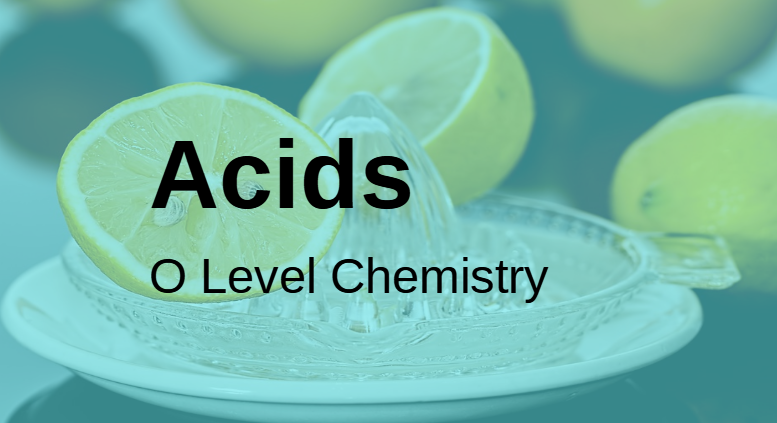# Acids for O Level ChemistryIn this post, let’s talk about acids for O Level Chemistry.

## What are acids – O Level Chemistry definition

In O Level Chemistry, students learn that an acid is a substance that dissociates to give hydrogen ions (H+) in water.

In the example below, hydrogen chloride (HCl) dissolves and dissociates in water to give hydrogen ion. Hence, hydrochloric acid is an acid.

HCl (aq) –> H+(aq) + Cl(aq)

This is an Arrhenius Acid (do note that there are many definitions of acids, and the one that we learn in O level chemistry is the Arrhenius one).

## Properties of acids

Some common properties we are familiar with include acids have a sour taste, turn blue litmus paper red, and are corrosive.

## Common Acids in O Level Chemistry

Here are the common acids in O Level Chemistry:

Notice that all the acids ionizes or dissociates in water to give hydrogen ions in water? Hence they are acids.

## Weak vs Strong Acids

A weak acid is one that dissociates partially in water. E.g. most organic acids such as ethanoic acid. The reversible arrow sign () indicates partial dissociation.

CH3COOH (aq) H+(aq) + CH3COO(aq)

A strong acid is one that dissociates completely in water e.g. hydrochloric acid, sulfuric acid, nitric acid. The arrow (–>) indicates complete dissociation.

HCl (aq) –> H+(aq) + Cl(aq)

## Acid reactions

Here are the acid reactions to know for O Level Chemistry:

1. Acids react with reactive metals (i.e. metals above hydrogen in the reactivity series) to give salt and hydrogen

word equation: acid + metal –> salt + hydrogen

2. Acids react with bases to give salt and water

word equation: acid + base –> salt + water

3. Acids react with carbonates to give salt, carbon dioxide and water

word equation: acid + carbonate –> salt + carbon dioxide + water

## More on acids, bases and salts

Want a simplified course that will teach you the basics of acids, bases and salts and more? Check out our course on acids, bases and salts here.

We also have courses on other topics. All these are on-demand courses where students can watch them anytime, anywhere and learn at their own pace.

In this course, students will learn the chapters on atomic structure, chemical bonding, elements, molecules and compounds, and also the fundamentals of chemistry – writing equations, and chemical formulae. Check out this course here.

In this course, students will learn acids, bases and salts, qualitative analysis, metals, periodic table and ammonia. I also share a way of remembering the metals in the reactivity series in this course. You can get this on-demand course here.

Questions on mole concept and stoichiometry can be combined with any other chapter. In this course, I will go through with you step- by-step all the concepts you need to know for mole concept and stoichiometry. You can get this on-demand course here.

These topics involve applying concepts learnt to scenario-based questions. In our on-demand course on the rate of reaction and heat of reaction, we go through the concepts and how to apply them to questions. You can get this on-demand course here.

Learn redox reactions, and electrolysis and electric cell n this on-demand course here. Most schools cover electrolysis last, so if you want to learn ahead of your school, check out our course here.

In O Level Chemistry, students are expected to know the properties, and reactions of alkanes, alkenes, alcohols, carboxylic acids, esters and polymers (addition and condensation polymers). We talk about them in detail in our on-demand course on O Level Organic Chemistry. You can get this on-demand course here.

error: Content is protected !!